# Chapter 5 unit f 001

30 Jan 2015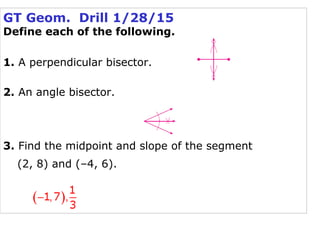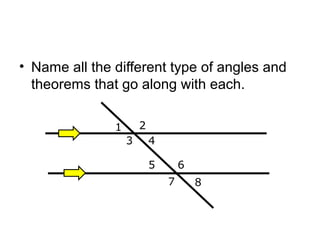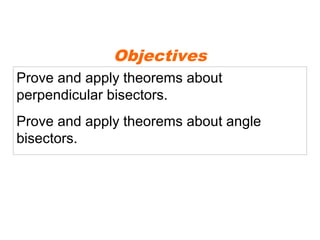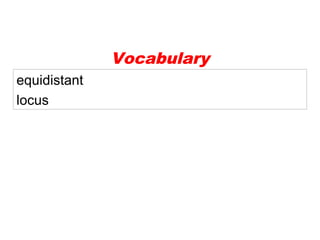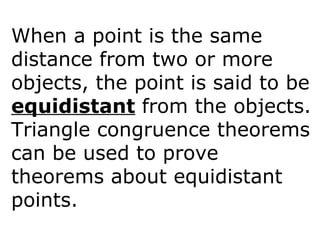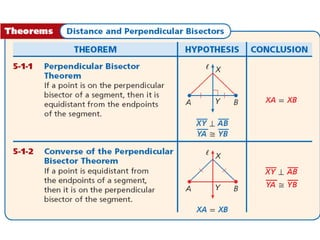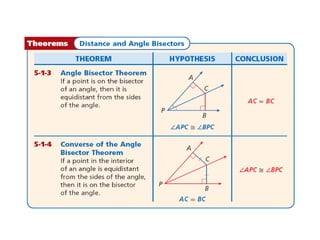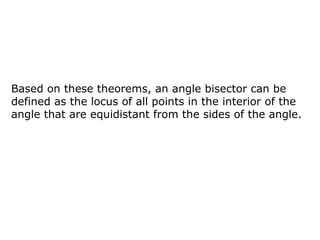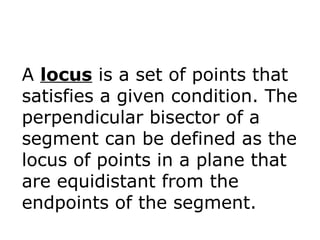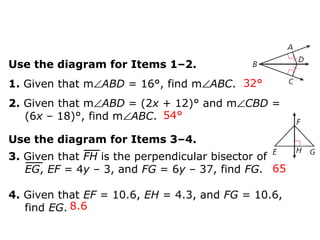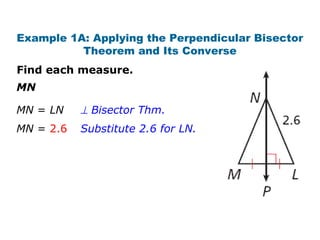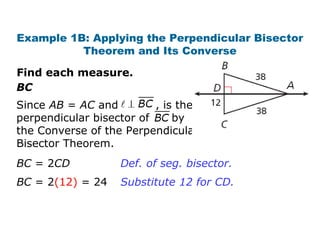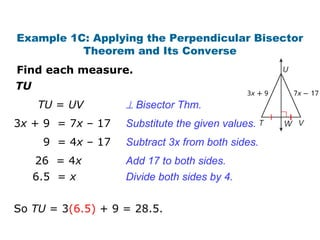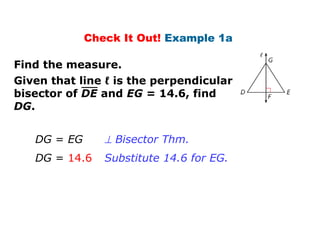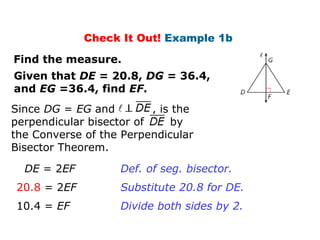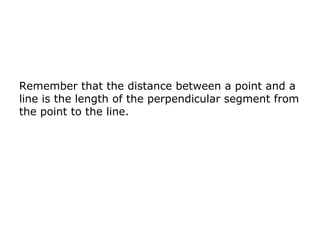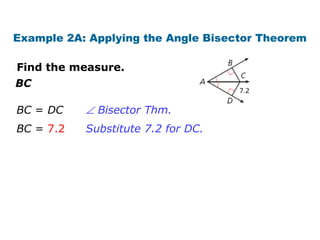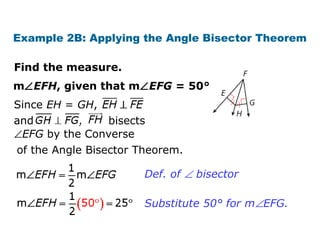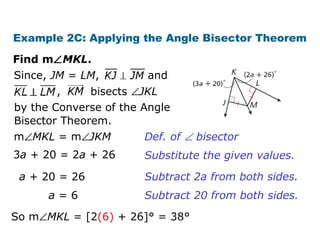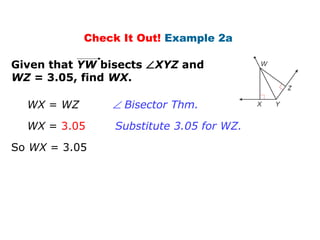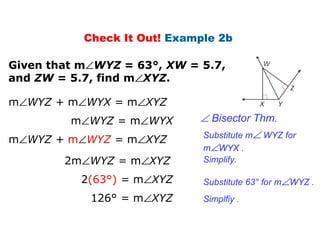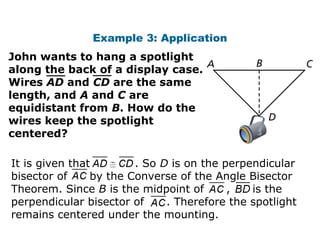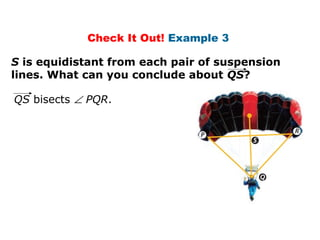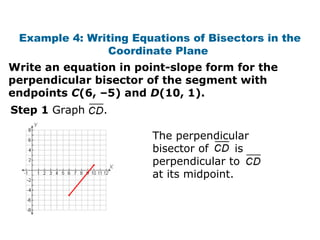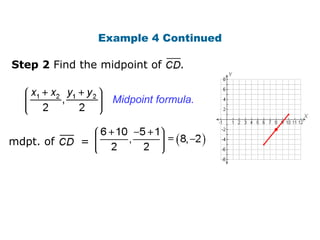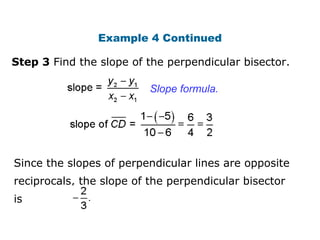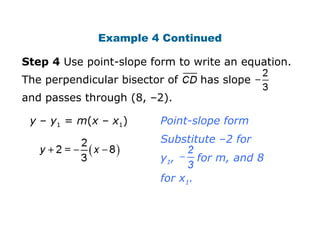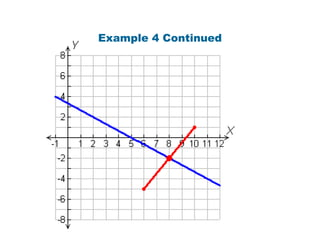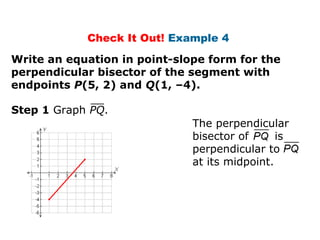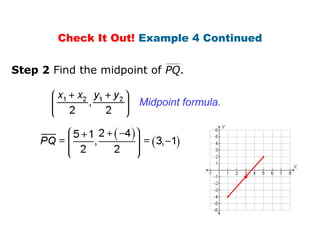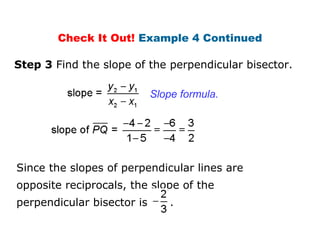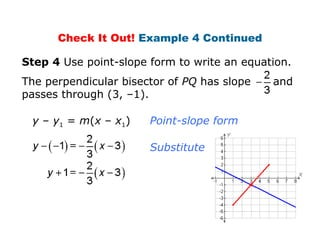1 sur 36

### Chapter 5 unit f 001

• 1. GT Geom. Drill 1/28/15 Define each of the following. 1. A perpendicular bisector. 2. An angle bisector. 3. Find the midpoint and slope of the segment (2, 8) and (–4, 6).
• 2. • Name all the different type of angles and theorems that go along with each. 1 3 2 5 4 6 7 8
• 3. Prove and apply theorems about perpendicular bisectors. Prove and apply theorems about angle bisectors. Objectives
• 5. When a point is the same distance from two or more objects, the point is said to be equidistant from the objects. Triangle congruence theorems can be used to prove theorems about equidistant points.
• 8. Based on these theorems, an angle bisector can be defined as the locus of all points in the interior of the angle that are equidistant from the sides of the angle.
• 9. A locus is a set of points that satisfies a given condition. The perpendicular bisector of a segment can be defined as the locus of points in a plane that are equidistant from the endpoints of the segment.
• 10. Use the diagram for Items 1–2. 1. Given that m∠ABD = 16°, find m∠ABC. 2. Given that m∠ABD = (2x + 12)° and m∠CBD = (6x – 18)°, find m∠ABC. 32° 54° 65 8.6 Use the diagram for Items 3–4. 3. Given that FH is the perpendicular bisector of EG, EF = 4y – 3, and FG = 6y – 37, find FG. 4. Given that EF = 10.6, EH = 4.3, and FG = 10.6, find EG.
• 11. Example 1A: Applying the Perpendicular Bisector Theorem and Its Converse Find each measure. MN MN = LN MN = 2.6 ⊥ Bisector Thm. Substitute 2.6 for LN.
• 12. Example 1B: Applying the Perpendicular Bisector Theorem and Its Converse Find each measure. BC Since AB = AC and , is the perpendicular bisector of by the Converse of the Perpendicular Bisector Theorem. BC = 2CD BC = 2(12) = 24 Def. of seg. bisector. Substitute 12 for CD.
• 13. Example 1C: Applying the Perpendicular Bisector Theorem and Its Converse TU Find each measure. So TU = 3(6.5) + 9 = 28.5. TU = UV ⊥ Bisector Thm. 3x + 9 = 7x – 17 9 = 4x – 17 26 = 4x 6.5 = x Subtract 3x from both sides. Add 17 to both sides. Divide both sides by 4. Substitute the given values.
• 14. Check It Out! Example 1a Find the measure. Given that line ℓ is the perpendicular bisector of DE and EG = 14.6, find DG. DG = EG DG = 14.6 ⊥ Bisector Thm. Substitute 14.6 for EG.
• 15. Check It Out! Example 1b Given that DE = 20.8, DG = 36.4, and EG =36.4, find EF. Find the measure. DE = 2EF 20.8 = 2EF Def. of seg. bisector. Substitute 20.8 for DE. Since DG = EG and , is the perpendicular bisector of by the Converse of the Perpendicular Bisector Theorem. 10.4 = EF Divide both sides by 2.
• 16. Remember that the distance between a point and a line is the length of the perpendicular segment from the point to the line.
• 17. Example 2A: Applying the Angle Bisector Theorem Find the measure. BC BC = DC BC = 7.2 ∠ Bisector Thm. Substitute 7.2 for DC.
• 18. Example 2B: Applying the Angle Bisector Theorem Find the measure. m∠EFH, given that m∠EFG = 50°. Since EH = GH, and , bisects ∠EFG by the Converse of the Angle Bisector Theorem. Def. of ∠ bisector Substitute 50° for m∠EFG.
• 19. Example 2C: Applying the Angle Bisector Theorem Find m∠MKL. , bisects ∠JKL Since, JM = LM, and by the Converse of the Angle Bisector Theorem. m∠MKL = m∠JKM 3a + 20 = 2a + 26 a + 20 = 26 a = 6 Def. of ∠ bisector Substitute the given values. Subtract 2a from both sides. Subtract 20 from both sides. So m∠MKL = [2(6) + 26]° = 38°
• 20. Check It Out! Example 2a Given that YW bisects ∠XYZ and WZ = 3.05, find WX. WX = WZ So WX = 3.05 WX = 3.05 ∠ Bisector Thm. Substitute 3.05 for WZ.
• 21. Check It Out! Example 2b Given that m∠WYZ = 63°, XW = 5.7, and ZW = 5.7, find m∠XYZ. m∠WYZ = m∠WYX m∠WYZ + m∠WYX = m∠XYZ m∠WYZ + m∠WYZ = m∠XYZ 2(63°) = m∠XYZ 126° = m∠XYZ ∠ Bisector Thm. Substitute m∠ WYZ for m∠WYX . 2m∠WYZ = m∠XYZ Simplify. Substitute 63° for m∠WYZ . Simplfiy .
• 22. Example 3: Application John wants to hang a spotlight along the back of a display case. Wires AD and CD are the same length, and A and C are equidistant from B. How do the wires keep the spotlight centered? It is given that . So D is on the perpendicular bisector of by the Converse of the Angle Bisector Theorem. Since B is the midpoint of , is the perpendicular bisector of . Therefore the spotlight remains centered under the mounting.
• 23. Check It Out! Example 3 S is equidistant from each pair of suspension lines. What can you conclude about QS? QS bisects ∠ PQR.
• 24. Example 4: Writing Equations of Bisectors in the Coordinate Plane Write an equation in point-slope form for the perpendicular bisector of the segment with endpoints C(6, –5) and D(10, 1). Step 1 Graph . The perpendicular bisector of is perpendicular to at its midpoint.
• 25. Example 4 Continued Step 2 Find the midpoint of . Midpoint formula. mdpt. of =
• 26. Step 3 Find the slope of the perpendicular bisector. Example 4 Continued Slope formula. Since the slopes of perpendicular lines are opposite reciprocals, the slope of the perpendicular bisector is
• 27. Example 4 Continued Step 4 Use point-slope form to write an equation. The perpendicular bisector of has slope and passes through (8, –2). y – y1 = m(x – x1) Point-slope form Substitute –2 for y1, for m, and 8 for x1.
• 29. Check It Out! Example 4 Write an equation in point-slope form for the perpendicular bisector of the segment with endpoints P(5, 2) and Q(1, –4). Step 1 Graph PQ. The perpendicular bisector of is perpendicular to at its midpoint.
• 30. Check It Out! Example 4 Continued Step 2 Find the midpoint of PQ. Midpoint formula.
• 31. Step 3 Find the slope of the perpendicular bisector. Check It Out! Example 4 Continued Slope formula. Since the slopes of perpendicular lines are opposite reciprocals, the slope of the perpendicular bisector is .
• 32. Substitute. Check It Out! Example 4 Continued Step 4 Use point-slope form to write an equation. The perpendicular bisector of PQ has slope and passes through (3, –1). y – y1 = m(x – x1) Point-slope form
• 34. Lesson Quiz: Part II 5. Write an equation in point-slope form for the perpendicular bisector of the segment with endpoints X(7, 9) and Y(–3, 5) .